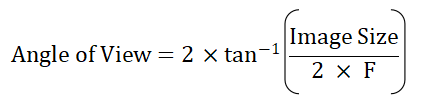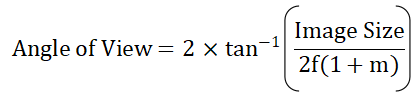# Focal Length Calculator (Mirror)

This is an online calculator to find the focal length of a mirror. Just enter the object size, image size, and object distance to get the magnification, angle of view, and focal length value.

• mm
• mm
• mm

### Result

• Magnification
• Focal Length of the Mirror
mm
• Effective Focal Length
mm
• Angle of View
Degrees

Mirror is any surface that redirects light rays, thereby forming an image of the object. They may be plane or curved. Image formation by mirrors involves only the law of reflection.

Focal Length of a mirror is the distance between the vertex of the mirror and the focal point.Consider the image formation by a spherical mirror. Figure shows a concave mirror with a radius of curvature R. The center of curvature of the surface is at C and the vertex of the mirror is at P. The line OP is the optic axis. Point O is an object lying on the optic axis and we assume that the distance OP is greater than R.

The ray from O at the angle α with the axis strikes the mirror at Q and is reflected. The incident and reflected rays make equal angle θ with the normal CQ (law of reflection). A second ray from O, passing through C strikes the mirror normally and is reflected back on itself. The two reflected rays intersect at I forming a real image of O.

In a triangle, the exterior angle is equal to the sum of the two opposite interior angles. Applying this in ∆OQC,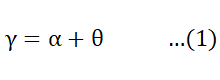Similarly in ∆OQL,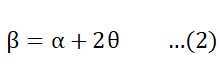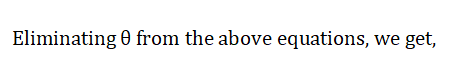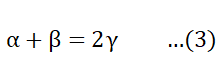Let h be the height of the point Q above the optic axis and let δ be the short distance BP.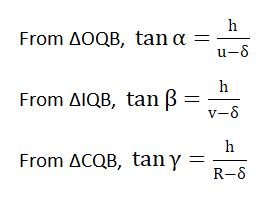According to paraxial approximation, for small angles, α, β, Ƴ, tan α can be replaced by α and so on. So we get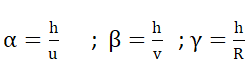Substituting these values in (3), we get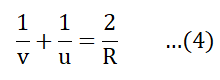This is known as the Spherical Mirror Equation.

If the object is taken at a very large distance from the mirror (u=∞), then the incoming rays are almost parallel, then the above equation becomes,The point at which the incident parallel rays converge is called the focal point and the distance from the vertex to the focal point is called the focal length denoted by f. So focal length is the image distance when the object is at infinity. Thus, v = f and f = R/2

Thus (4) can be rewritten as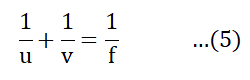This is known as the Gauss formula for Spherical Mirrors.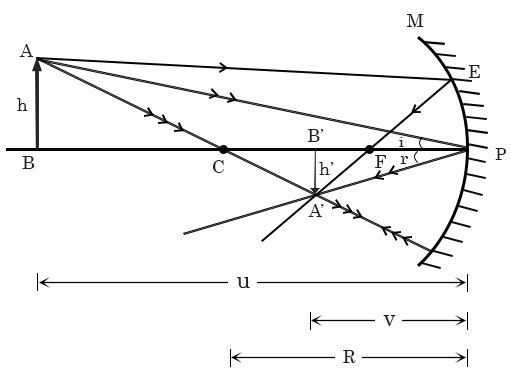The ratio of dimensions of the final image formed by an optical system to the corresponding dimension of the object is defined as the magnification. Here the object and image have different sizes and they have opposite orientations. Then from the figure, we can write,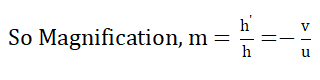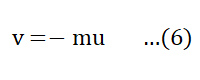Thus rewriting (5) using (6), we get,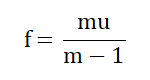In the case of a mirror, where the mirror-to-object distance is comparable to the focal length, we need to consider the magnification factor, hence the effective focal length can be written as,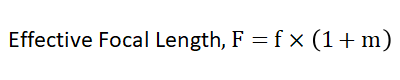Angle of View is the angle subtended at the image of the eye by the mirror. It is defined in terms of the image size and the effective focal length,